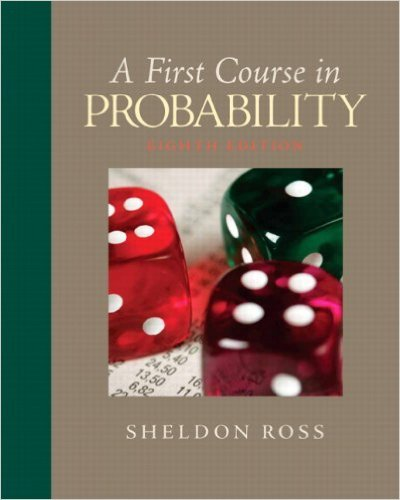> > > Chapter 8

# Solutions for Chapter 8: First Course in Probability 8th Edition## Full solutions for First Course in Probability | 8th Edition

ISBN: 9780136033134Solutions for Chapter 8

Solutions for Chapter 8
4 5 0 235 Reviews
15
3
##### ISBN: 9780136033134

First Course in Probability was written by Sieva Kozinsky and is associated to the ISBN: 9780136033134. Since 23 problems in chapter 8 have been answered, more than 3273 students have viewed full step-by-step solutions from this chapter. Chapter 8 includes 23 full step-by-step solutions. This textbook survival guide was created for the textbook: First Course in Probability, edition: 8. This expansive textbook survival guide covers the following chapters and their solutions.

Key Statistics Terms and definitions covered in this textbook
• Arithmetic mean

The arithmetic mean of a set of numbers x1 , x2 ,…, xn is their sum divided by the number of observations, or ( / )1 1 n xi t n ? = . The arithmetic mean is usually denoted by x , and is often called the average

• Average run length, or ARL

The average number of samples taken in a process monitoring or inspection scheme until the scheme signals that the process is operating at a level different from the level in which it began.

• Cause-and-effect diagram

A chart used to organize the various potential causes of a problem. Also called a ishbone diagram.

• Central tendency

The tendency of data to cluster around some value. Central tendency is usually expressed by a measure of location such as the mean, median, or mode.

• Conditional probability

The probability of an event given that the random experiment produces an outcome in another event.

• Continuous uniform random variable

A continuous random variable with range of a inite interval and a constant probability density function.

• Control chart

A graphical display used to monitor a process. It usually consists of a horizontal center line corresponding to the in-control value of the parameter that is being monitored and lower and upper control limits. The control limits are determined by statistical criteria and are not arbitrary, nor are they related to speciication limits. If sample points fall within the control limits, the process is said to be in-control, or free from assignable causes. Points beyond the control limits indicate an out-of-control process; that is, assignable causes are likely present. This signals the need to ind and remove the assignable causes.

• Covariance

A measure of association between two random variables obtained as the expected value of the product of the two random variables around their means; that is, Cov(X Y, ) [( )( )] =? ? E X Y ? ? X Y .

• Crossed factors

Another name for factors that are arranged in a factorial experiment.

• Cumulative distribution function

For a random variable X, the function of X deined as PX x ( ) ? that is used to specify the probability distribution.

• Deming’s 14 points.

A management philosophy promoted by W. Edwards Deming that emphasizes the importance of change and quality

• Design matrix

A matrix that provides the tests that are to be conducted in an experiment.

• Eficiency

A concept in parameter estimation that uses the variances of different estimators; essentially, an estimator is more eficient than another estimator if it has smaller variance. When estimators are biased, the concept requires modiication.

• Enumerative study

A study in which a sample from a population is used to make inference to the population. See Analytic study

• First-order model

A model that contains only irstorder terms. For example, the irst-order response surface model in two variables is y xx = + ?? ? ? 0 11 2 2 + + . A irst-order model is also called a main effects model

• Fractional factorial experiment

A type of factorial experiment in which not all possible treatment combinations are run. This is usually done to reduce the size of an experiment with several factors.

• Frequency distribution

An arrangement of the frequencies of observations in a sample or population according to the values that the observations take on

• Gamma random variable

A random variable that generalizes an Erlang random variable to noninteger values of the parameter r

• Gaussian distribution

Another name for the normal distribution, based on the strong connection of Karl F. Gauss to the normal distribution; often used in physics and electrical engineering applications

• Goodness of fit

In general, the agreement of a set of observed values and a set of theoretical values that depend on some hypothesis. The term is often used in itting a theoretical distribution to a set of observations.

×

I don't want to reset my password

Need help? Contact support

Need an Account? Is not associated with an account
We're here to help

×

I don't want to reset my password

Need help? Contact support

Need an Account? Is not associated with an account
We're here to help